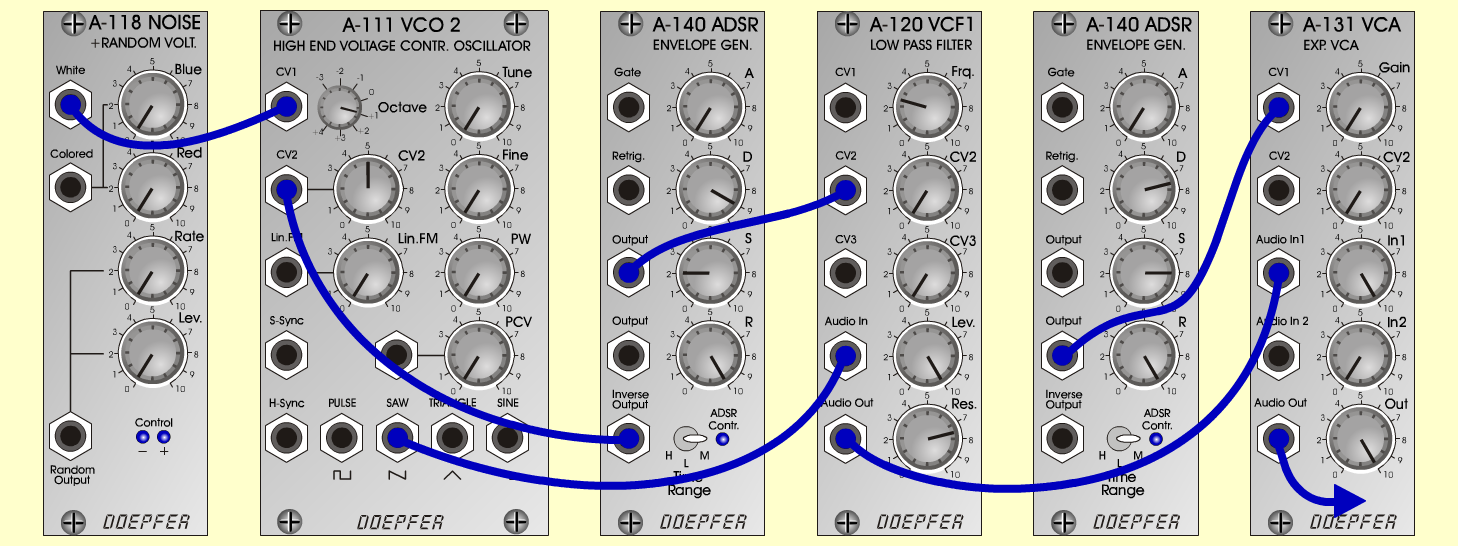ThunderN a t u r eD o e p f e r A - 100 Connections: Settings: A-118 (White)  <=>  A-119 (Asym In) A-119 (Audio Out)  <=>  A-111 (CV1) A-111 (Saw)  <=>  A-120 (Audio In) A-120 (Audio Out)  <=>  A-131 (Audio In 1) A-140/1 (Output)  <=>  A-120 (CV2) A-140/1 (Inverse Output)  <=>  A-111 (CV2) A-140/2 (Output)  <=>  A-131 (CV1) KB (Gate)  <=> A-140/1+2 (Gate) A-111 (Octave = +1, Tune = 0, Fine = 0, CV 2 = 5)A-120 (Freq = 2.5, Lev = 10, Res = 7.5) A-131 (Gain = 0, Audio In 1 = 10,  Audio Out = 10) A-140/1 (A = 0, D = 9, S = 2, R = 10, Range = M) A-140/2 (A = 0, D = 7.5, S = 8, R = 10, Range = M) Notes A-111: The modul A-110 is also possible. (See german magazin KEYBOARDS: Basic Thunder / Soundforum-Synthesizer) Josef MuellerSound samples Bad weather (with Stormy & Rain) Thunder long Thunder short